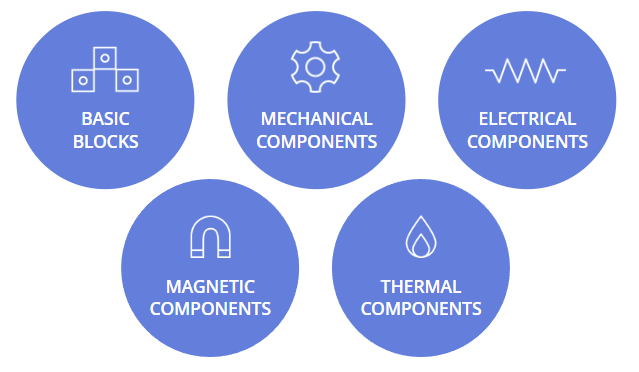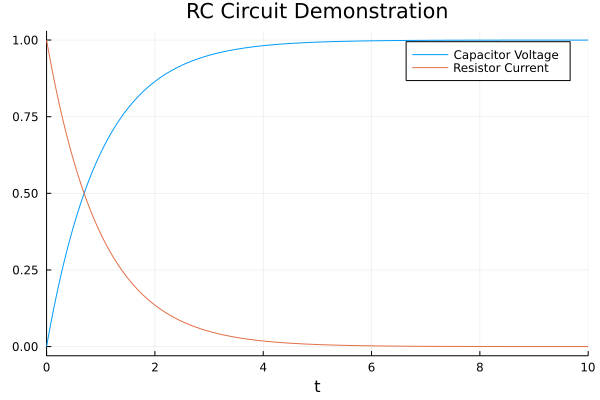## ModelingToolkitStandardLibrary.jl

A standard library of components to model the world and beyond
Popularity
76 Stars
Updated Last
4 Months Ago
Started In
November 2021

# ModelingToolkitStandardLibrary.jl

The ModelingToolkit Standard Library is a standard library of components to model the world and beyond.## Installation

Assuming that you already have Julia correctly installed, it suffices to import ModelingToolkitStandardLibrary.jl in the standard way:

```import Pkg;

## Tutorials and Documentation

For information on using the package, see the stable documentation. Use the in-development documentation for the version of the documentation, which contains the unreleased features.

## Libraries

The following are the constituant libraries of the ModelingToolkit Standard Library.

## Example

The following is the RC Circuit Demonstration:

```using ModelingToolkit, OrdinaryDiffEq, Plots
using ModelingToolkitStandardLibrary.Electrical
using ModelingToolkitStandardLibrary.Blocks: Constant

R = 1.0
C = 1.0
V = 1.0
@variables t
@named resistor = Resistor(R = R)
@named capacitor = Capacitor(C = C)
@named source = Voltage()
@named constant = Constant(k = V)
@named ground = Ground()

rc_eqs = [connect(constant.output, source.V)
connect(source.p, resistor.p)
connect(resistor.n, capacitor.p)
connect(capacitor.n, source.n, ground.g)]

@named rc_model = ODESystem(rc_eqs, t,
systems = [resistor, capacitor, constant, source, ground])
sys = structural_simplify(rc_model)
prob = ODEProblem(sys, Pair[], (0, 10.0))
sol = solve(prob, Tsit5())
plot(sol, idxs = [capacitor.v, resistor.i],
title = "RC Circuit Demonstration",
labels = ["Capacitor Voltage" "Resistor Current"])
savefig("plot.png")```### Required Packages

View all packages